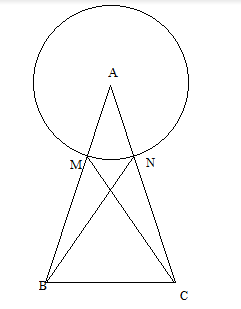QuestionAnswers

# ABC is an equilateral triangle. A circle is drawn with centre A so that it cuts AB and AC at points M and N respectively. Prove that BN= CM.

Hint: In this question, we will first draw the diagram. After this, we use the concept that the radius of the circle is equal, we will find MB and NC. Now, we will apply the SAS congruence rule in triangle MBC and triangle NBC.

Let us first draw the diagram of the question:A is the centre of the circle and AM and AN are the radius of the circle.
$\therefore$ AM = AN (1)
It is given that triangle ABC is an equilateral triangle. So, AB = BC = CA and $\angle$ MBC = $\angle$ NCB = $\angle$ BAC = ${60^0}$
Also, AM+MB = AN+NC
From equation 1, we get:
MB = NC.
Now, in $\vartriangle MBC{\text{ and }}\vartriangle {\text{NBC}}$ , we have:
MB = NC
$\angle$ MBC= $\angle$ NCB = ${60^0}$
And BC = BC
SO, by SAS rule of congruence, we can say that:
$\vartriangle MBC \cong \vartriangle NBC$
And by c.p.c.t., we can say that CM = BN.
Therefore, we have proved that CM= BN.

Note: In this type of question, you should first draw the diagram so that things gets clear for further calculation. You should remember the different rules of congruency which are given below:
1. SSS rule – All sides of one triangle are equal to all corresponding sides of another triangle.
2.SAS- Two sides and one angle formed between these two sides of one triangle is equal to that other triangle.
3. ASA- one side and two angles formed on this side of one triangle is equal to that of the other triangle.
4. RHS- This is applicable for the right triangle. Hypotenuse and one other side of one triangle is equal to that of another triangle.